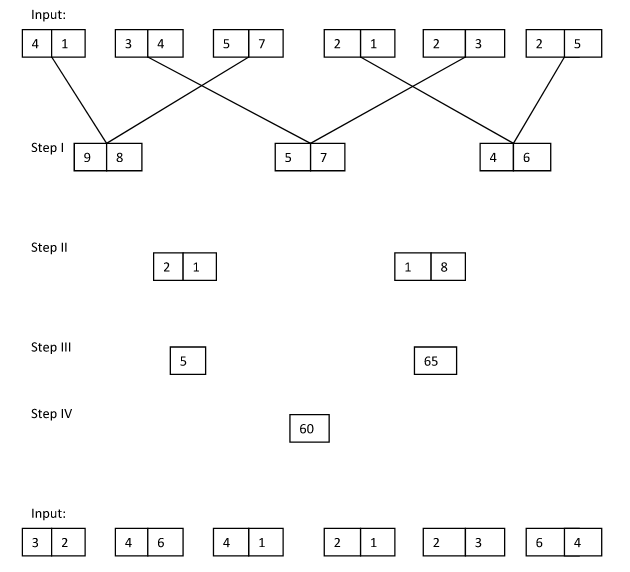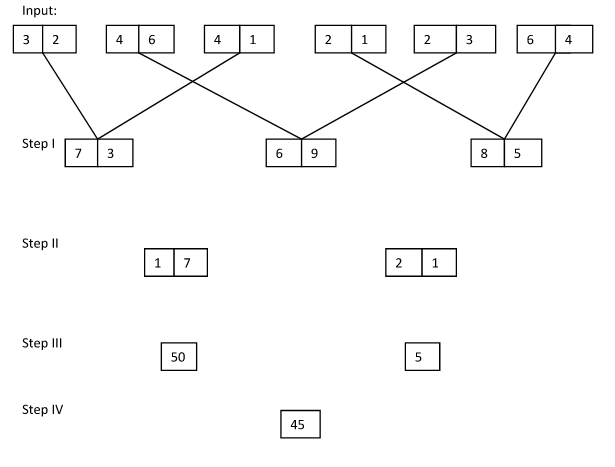# Logical Reasoning (Miscellaneous) Clerk / PO 2018 – Day-21

## Crack SBI Clerk / Canara Bank PO 2018 – Logical Reasoning (Miscellaneous) Day-21:

Dear Readers, Reasoning Section play a vital role in Banking and all other competitive exams. This year 2018 was started with three wonderful opportunities; Canara Bank PO Exam and Most Awaited SBI Clerk 2018. To enrich your preparation here we have providing new series of Practice Questions on Reasoning Ability – Miscellaneous. Candidates those who are going to appear in Bank Exams 2018 can practice these questions daily and make your preparation effective.

[WpProQuiz 1393]

## Daily Practice Test Schedule | Good Luck

 Topic Daily Publishing Time Daily News Papers & Editorials 8.00 AM Current Affairs Quiz 9.00 AM Logical Reasoning 10.00 AM Quantitative Aptitude “20-20” 11.00 AM Vocabulary (Based on The Hindu) 12.00 PM Static GK Quiz 1.00 PM English Language “20-20” 2.00 PM Banking Awareness Quiz 3.00 PM Reasoning Puzzles & Seating 4.00 PM Daily Current Affairs Updates 5.00 PM Data Interpretation / Application Sums (Topic Wise) 6.00 PM Reasoning Ability “20-20” 7.00 PM English Language (New Pattern Questions) 8.00 PM General / Financial Awareness Quiz 9.00 PM

Directions (1-5): Study the following information carefully and answer the given questions.

A number arrangement machine arranges two digit numbers into a typical manner. Each step gives output taking input from the previous step. The following is an illustration of Input and rearrangement. Using the illustration answer the question given below.1. If each digit in the step II is doubled and then added, then what will be the final sum?
1. 28
2. 34
3. 22
4. 46
5. None of these
1. Which of the following value to add with final input to make the final input value as perfect square?
1. 14
2. 9
3. 7
4. 4
5. None of these
1. Which of the following combination represent the first digit of the third number and second digit of the first number in step I of the given input?
1. 9, 7
2. 6, 5
3. 8, 9
4. 5, 6
5. None of these
1. Which of the following represent the difference between the first digit of the second number and second digit of the first number in step II?
1. 3
2. 5
3. 1
4. 4
5. None of these
1. What is the multiplication of two numbers obtained in step III?
1. 250
2. 175
3. 225
4. 325
5. None of these

6) In a certain code ‘FORT’ is written as ‘5914’ and ‘MOCK’ is written as ‘3926’. How is ‘TORM’ written in that code?

1. 4913
2. 4193
3. 9413
4. 3149
5. None of these

7) How many meaningful English words can be made with the letters ATME using each letter only once in each word?

a) None

b) One

c) Two

d) Three

e) More than three

8) Four of the following five are alike in a certain way and so form a group. Which is the one that does not belong to that group?

1. 49
2. 65
3. 39
4. 31
5. 25

9) In a certain code language, ‘go and come’ is written as ‘na ta ka’ and ‘black and white’ is written as ‘pa ma ta’. How is ‘go’ written in that code language?

1. na
2. ka
3. pa
4. na or ka
5. None

10) If each of the odd digits in the number 4587631 is changed to the next even digit and the even digits are kept unchanged, how many digits will appear only once in the new number?

1. None
2. One
3. Two
4. Three
5. More than three

Direction (1-5):Step I – First digit add with the first digit and second digit add with the second digit

Step II –

First number  = Sum of second digit of all three numbers

= 3 + 5 + 9 = 17

Second number =  Sum of first digit of all three numbers

= 7 + 6 + 8 = 21

Step III – Sum of square of both digits

First number – 7^2 + 1^2 = 49 + 1 = 50

Second number – 2^2 + 1^2 = 4 + 1 = 5

Step IV – Difference of both numbers

FORT – 5 9 1 4

MOCK – 3 9 2 6

TORM – 4913

7) Answer  e) More than three

MEAT, MATE, TEAM ,TAME

Except option (d) all four are not  prime numbers.

9) Answer d) na or ka

and = ta

go/come = na or ka

If odd digits changed to even digits

4 5 8 7 6 3 1

4 6 8 8 6 4 2